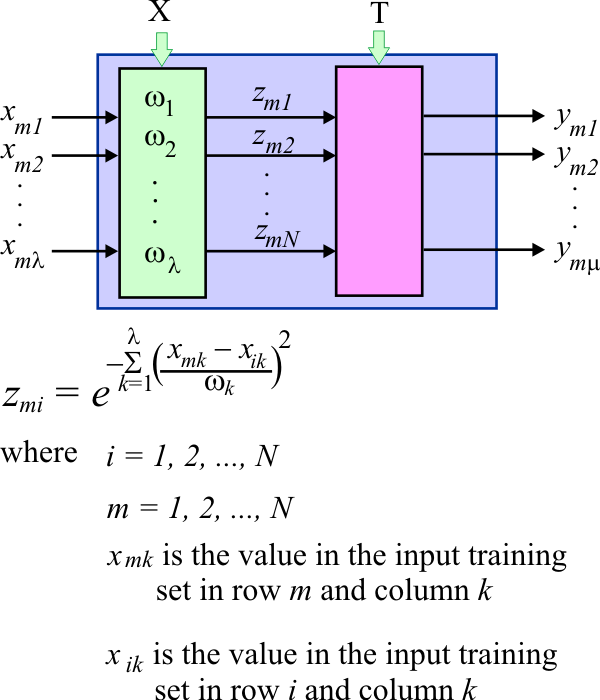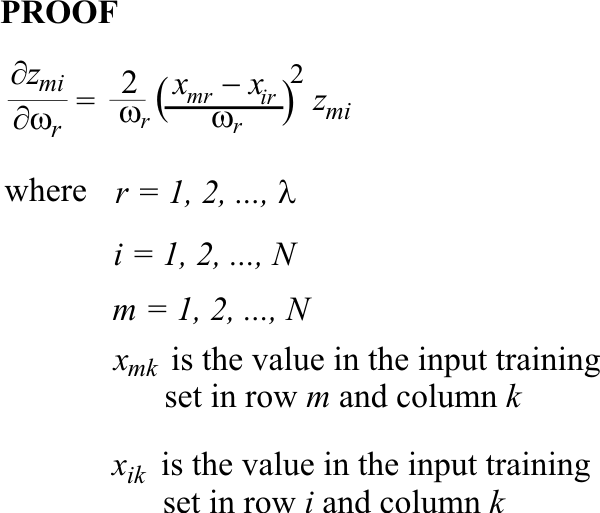# Partial Derivative of z

 Tip The partial derivatives of z are very important because they are used to find the PNN weights during training. The figure below shows the output of a PNN when the m input case is applied.Problem 1 Proof the zmi is equal to one when m is equal to i.

 Problem 2 Proof that the partial derivative of zmi with respect to the weight wr is given as follows.